# Hydrogen Fine Structure

When the familiar red spectral line of the hydrogen spectrum is examined at very high resolution, it is found to be a closely-spaced doublet. This splitting is called fine structure and was one of the first experimental evidences for electron spin.The small splitting of the spectral line is attributed to an interaction between the electron spin S and the orbital angular momentum L. It is called the spin-orbit interaction.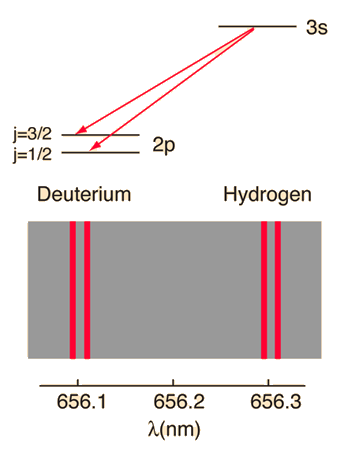The familiar red H-alpha line of hydrogen is a single line according to the Bohr theory. The straight application of the Schrodinger equation to the hydrogen atom gives the same result. If you calculate the wavelength of this line using the energy expression from the Bohr theory, you get 656.11 nm for hydrogen, treating the nucleus as a fixed center. If you use the reduced mass, you get 656.47 nm for hydrogen and 656.29 nm for deuterium. The difference between the hydrogen and deuterium lines is about 0.2 nm and the splitting of each of them is about 0.016 nm, corresponding to an energy difference of about 0.000045 eV. This corresponds to an internal magnetic field on the electron of about 0.4 Tesla.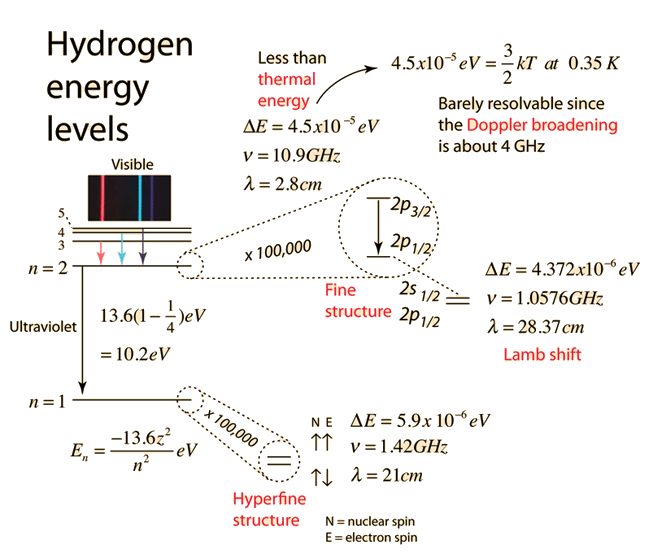Active illustration: click for more detail

 Examination of fine structure by saturation spectroscopy
Index

Schrodinger equation concepts

Hydrogen concepts

Atomic structure concepts

Reference
Rohlf
Sec 8.6

 HyperPhysics***** Quantum Physics R Nave
Go Back

# Spin-Orbit Interaction

The energy levels of atomic electrons are affected by the interaction between the electron spin magnetic moment and the orbital angular momentum of the electron. It can be visualized as a magnetic field caused by the electron's orbital motion interacting with the spin magnetic moment. This effective magnetic field can be expressed in terms of the electron orbital angular momentum. The interaction energy is that of a magnetic dipole in a magnetic field and takes the formWhen atomic spectral lines are split by the application of an external magnetic field, it is called the Zeeman effect. The spin-orbit interaction is also a magnetic interaction, but with the magnetic field generated by the orbital motion of an electron within the atom itself. It has been described as an "internal Zeeman effect". The standard example is the hydrogen fine structure.

 Hydrogen fine structure
 Spin-orbit splitting in sodium
Index

Schrodinger equation concepts

Atomic structure concepts

 HyperPhysics***** Quantum Physics R Nave
Go Back

# Effective Magnetic Field of Orbit

The classical magnetic field in the electron frame of reference arising from the orbital motion is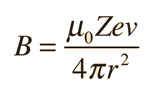Using classical physics and assuming a circular orbit, the angular momentum is L = mrv, so this field can be expressed in terms of the orbital angular momentum L:This is making use of the Bohr model where L=nh/2π . For a hydrogen electron in a 2p state at a radius of 4x the Bohr radius, this translates to a magnetic field of about 0.03 Tesla.

The magnetic field exerted on the orbiting electron as a result of the orbit produces an energy by the spin-orbit interaction. This energy contribution depends upon the relative orientation of its orbital and spin angular momentum. The magnetic field at the electron is in the direction of the orbital angular momentum. The energy contribution can be expressed asWith the two orientations of spin, the energy separation ΔE can be expressed aswhere α is the fine structure constant:Considering the example of the fine structure of the n=2 hydrogen level shown above, that substitution with the approximation that the radius = a0n2 yields the value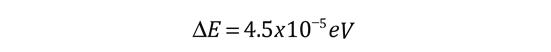Index

Schrodinger equation concepts

Blatt
Modern Physics Sec 9.3

 HyperPhysics***** Quantum Physics R Nave
Go Back

# Magnetic Field in Electron Frame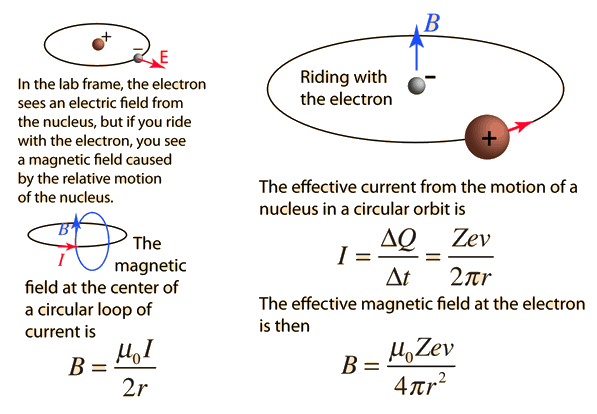Magnetic field of current loop Express in terms of angular momentum
Index

Schrodinger equation concepts

 HyperPhysics***** Quantum Physics R Nave
Go Back